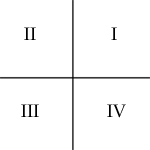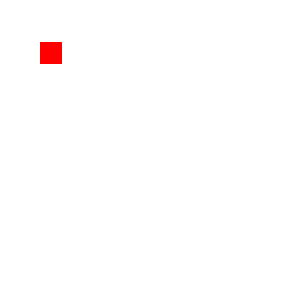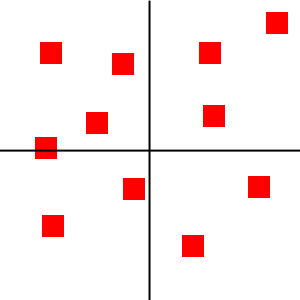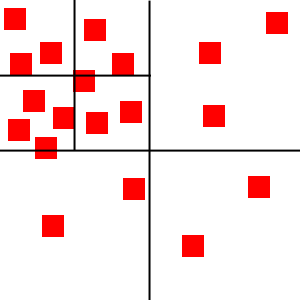# Quick Tip: Use Quadtrees to Detect Likely Collisions in 2D SpaceMany games require the use of collision detection algorithms to determine when two objects have collided, but these algorithms are often expensive operations and can greatly slow down a game. In this article we'll learn about quadtrees, and how we can use them to speed up collision detection by skipping pairs of objects that are too far apart to collide.

Note: Although this tutorial is written using Java, you should be able to use the same techniques and concepts in almost any game development environment.

## Introduction

Collision detection is an essential part of most video games. Both in 2D and 3D games, detecting when two objects have collided is important as poor collision detection can lead to some very interesting results:

However, collision detection is also a very expensive operation. Let’s say there are 100 objects that need to be checked for collision. Comparing each pair of objects requires 10,000 operations - that’s a lot of checks!

One way to speed things up is to reduce the number of checks that have to be made. Two objects that are at opposite ends of the screen can not possibly collide, so there is no need to check for a collision between them. This is where a quadtree comes into play.

A quadtree is a data structure used to divide a 2D region into more manageable parts. It's an extended binary tree, but instead of two child nodes it has four.

In the images below, each image is a visual representation of the 2D space and the red squares represent objects. For the purposes of this article, subnodes will be labelled counter-clockwise as follows:When more objects are added to the quadtree, it will eventually split into four subnodes. Each object will then be put into one of these subnodes according to where it lies in the 2D space. Any object that cannot fully fit inside a node’s boundary will be placed in the parent node.Each subnode can continue subdividing as more objects are added.As you can see, each node only contains a few objects. We know then that, for instance, the objects in the top-left node cannot be colliding with the objects in the bottom-right node, so we don't need to run an expensive collision detection algorithm between such pairs.

Take a look at this JavaScript example to see a quadtree in action.

Implementing a quadtree is fairly simple. The following code is written in Java, but the same techniques can be used for most other programming languages. I’ll comment after each code snippet.

We’ll start off by creating the main Quadtree class. Below is the code for Quadtree.java.

 1 public class Quadtree {  2 3  private int MAX_OBJECTS = 10;  4  private int MAX_LEVELS = 5;  5 6  private int level;  7  private List objects;  8  private Rectangle bounds;  9  private Quadtree[] nodes;  10 11  /*  12  * Constructor  13  */  14  public Quadtree(int pLevel, Rectangle pBounds) {  15  level = pLevel;  16  objects = new ArrayList();  17  bounds = pBounds;  18  nodes = new Quadtree;  19  }  20 } 

The Quadtree class is straightforward. MAX_OBJECTS defines how many objects a node can hold before it splits and MAX_LEVELS defines the deepest level subnode. Level is the current node level (0 being the topmost node), bounds represents the 2D space that the node occupies, and nodes are the four subnodes.

In this example, the objects the quadtree will hold are Rectangles, but for your own quadtree it can be whatever you want.

Next, we’ll implement the five methods of a quadtree: clear, split, getIndex, insert, and retrieve.

 1 /*  2  * Clears the quadtree  3  */  4  public void clear() {  5  objects.clear();  6 7  for (int i = 0; i < nodes.length; i++) {  8  if (nodes[i] != null) {  9  nodes[i].clear();  10  nodes[i] = null;  11  }  12  }  13  } 

The clear method clears the quadtree by recursively clearing all objects from all nodes.

 1 /*  2  * Splits the node into 4 subnodes  3  */  4  private void split() {  5  int subWidth = (int)(bounds.getWidth() / 2);  6  int subHeight = (int)(bounds.getHeight() / 2);  7  int x = (int)bounds.getX();  8  int y = (int)bounds.getY();  9 10  nodes = new Quadtree(level+1, new Rectangle(x + subWidth, y, subWidth, subHeight));  11  nodes = new Quadtree(level+1, new Rectangle(x, y, subWidth, subHeight));  12  nodes = new Quadtree(level+1, new Rectangle(x, y + subHeight, subWidth, subHeight));  13  nodes = new Quadtree(level+1, new Rectangle(x + subWidth, y + subHeight, subWidth, subHeight));  14  } 

The split method splits the node into four subnodes by dividing the node into four equal parts and initializing the four subnodes with the new bounds.

 1 /*  2  * Determine which node the object belongs to. -1 means  3  * object cannot completely fit within a child node and is part  4  * of the parent node  5  */  6  private int getIndex(Rectangle pRect) {  7  int index = -1;  8  double verticalMidpoint = bounds.getX() + (bounds.getWidth() / 2);  9  double horizontalMidpoint = bounds.getY() + (bounds.getHeight() / 2);  10 11  // Object can completely fit within the top quadrants  12  boolean topQuadrant = (pRect.getY() < horizontalMidpoint && pRect.getY() + pRect.getHeight() < horizontalMidpoint);  13  // Object can completely fit within the bottom quadrants  14  boolean bottomQuadrant = (pRect.getY() > horizontalMidpoint);  15 16  // Object can completely fit within the left quadrants  17  if (pRect.getX() < verticalMidpoint && pRect.getX() + pRect.getWidth() < verticalMidpoint) {  18  if (topQuadrant) {  19  index = 1;  20  }  21  else if (bottomQuadrant) {  22  index = 2;  23  }  24  }  25  // Object can completely fit within the right quadrants  26  else if (pRect.getX() > verticalMidpoint) {  27  if (topQuadrant) {  28  index = 0;  29  }  30  else if (bottomQuadrant) {  31  index = 3;  32  }  33  }  34 35  return index;  36  } 

The getIndex method is a helper function of the quadtree. It determines where an object belongs in the quadtree by determining which node the object can fit into.

 1 /*  2  * Insert the object into the quadtree. If the node  3  * exceeds the capacity, it will split and add all  4  * objects to their corresponding nodes.  5  */  6  public void insert(Rectangle pRect) {  7  if (nodes != null) {  8  int index = getIndex(pRect);  9 10  if (index != -1) {  11  nodes[index].insert(pRect);  12 13  return;  14  }  15  }  16 17  objects.add(pRect);  18 19  if (objects.size() > MAX_OBJECTS && level < MAX_LEVELS) {  20  if (nodes == null) {  21  split();  22  }  23 24  int i = 0;  25  while (i < objects.size()) {  26  int index = getIndex(objects.get(i));  27  if (index != -1) {  28  nodes[index].insert(objects.remove(i));  29  }  30  else {  31  i++;  32  }  33  }  34  }  35  } 

The insert method is where everything comes together. The method first determines whether the node has any child nodes and tries to add the object there. If there are no child nodes or the object doesn’t fit in a child node, it adds the object to the parent node.

Once the object is added, it determines whether the node needs to split by checking if the current number of objects exceeds the max allowed objects. Splitting will cause the node to insert any object that can fit in a child node to be added to the child node; otherwise the object will stay in the parent node.

 1 /*  2  * Return all objects that could collide with the given object  3  */  4  public List retrieve(List returnObjects, Rectangle pRect) {  5  int index = getIndex(pRect);  6  if (index != -1 && nodes != null) {  7  nodes[index].retrieve(returnObjects, pRect);  8  }  9 10  returnObjects.addAll(objects);  11 12  return returnObjects;  13  } 

The final method of the quadtree is the retrieve method. It returns all objects in all nodes that the given object could potentially collide with. This method is what helps to reduce the number of pairs to check collision against.

## Using This for 2D Collision Detection

Now that we have a fully functional quadtree, it’s time to use it to help reduce the checks needed for collision detection.

In a typical game, you’ll start by creating the quadtree and passing the bounds of the screen.

 1 Quadtree quad = new Quadtree(0, new Rectangle(0,0,600,600)); 

At every frame, you’ll insert all objects into the quadtree by first clearing the quadtree then using the insert method for every object.

 1 quad.clear();  2 for (int i = 0; i < allObjects.size(); i++) {  3  quad.insert(allObjects.get(i));  4 } 

Once all objects have been inserted, you’ll go through each object and retrieve a list of objects it could possibly collide with. You'll then check for collisions between each object in the list and the initial object, using a collision detection algorithm.

 1 List returnObjects = new ArrayList();  2 for (int i = 0; i < allObjects.size(); i++) {  3  returnObjects.clear();  4  quad.retrieve(returnObjects, objects.get(i));  5 6  for (int x = 0; x < returnObjects.size(); x++) {  7  // Run collision detection algorithm between objects  8  }  9 } 

Note: Collision detection algorithms are beyond the scope of this tutorial. See this article for an example.

## Conclusion

Collision detection can be an expensive operation and can slow down the performance of your game. Quadtrees are one way you can help speed up collision detection and keep your game running at top speeds.